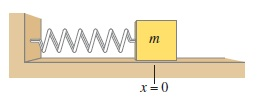# Problem: A mass on a spring in SHM has amplitude A and period T.Part AAt what point in the motion is the velocity zero and the acceleration zero simultaneously? a. x&gt;0 but x&lt;A. b. x=A c. x&lt;0. d. x=0. e. None of the above.

###### FREE Expert Solution

In this problem, we are applying the concept of the properties of various points during SHM.

97% (116 ratings)###### Problem Details

A mass on a spring in SHM has amplitude A and period T.

Part A

At what point in the motion is the velocity zero and the acceleration zero simultaneously?

a. x>0 but x<A.
b. x=A
c. x<0.
d. x=0.
e. None of the above.